## Counting in multiples of 6, 7, 8 and 9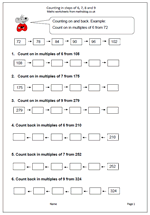A new target in the Maths Primary Framework for Year 4 is to count up in steps of 6, 7, and 9. This might sound easy and if the numbers are low there should not be a problem. However, counting up and down in steps of 6 or 7 starting from larger numbers can be tricky and might well involve a great deal of finger counting. Don’t be surprised at this as there is a lot of mathematical knowledge needed to successfully and quickly count up 7 from a number such as 196. It can be done by counting in single digits, or counting 4 to 200 and then 3, or even adding 10 and subtracting 3.

Counting in steps of 6,7,8 and 9

## Five times table in Year 2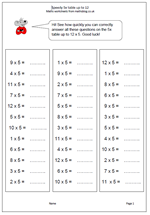Learning the five times table has now been placed firmly in the Year 2 Programme of Study and will prove quite a challenge for many children who find counting up to 100 hard, let alone reciting the 5 times table. To make it even more of a challenge the table now has to be learned up to 12 times 5 rather than just 10 times 5. There is a danger with this that children will learn the table ‘parrot fashion’ with little idea of what it means or how to use the knowledge.

There is, of course, a rhythm and pattern to the five times table, as alternate answers end in zero or 5. This makes it one of the easiest times tables to learn. My latest worksheet can be used as a timed practice, with three sets of 12 questions on the page.

Speedy 5x table

## Two times table up to 12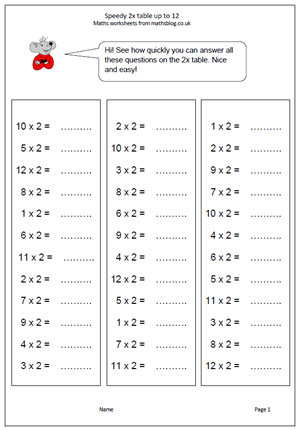In the new Maths Programme of Study children will be taught to recall and use the two times table up to twelve times two. There are many ways that times tables can be learned, but the end product will be the same; children will know the facts off by heart and be able to answer instantly. Note: knowing the two times table is not the same as being able to count up in twos. It is no good if a child has to start counting to work out what 9 x 2 is as it will take too long.

This page extends our current times table worksheets found in the Four Rules section and can be found in the Year 2 Calculating section.

Speedy 2x table up to 12

## New Maths Curriculum: Year 2 Statistics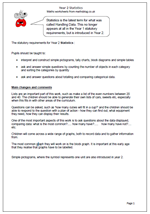Statistics is the latest term for what was called Handling Data. This no longer appears at all in the Year 1 statutory requirements, but is introduced in Year 2.

The statutory requirements for Year 2 Statistics :

Pupils should be taught to:

•    interpret and construct simple pictograms, tally charts, block diagrams and simple tables

•    ask and answer simple questions by counting the number of objects in each category and sorting the categories by quantity

Lists are an important part of this work, such as make a list of the even numbers between 20 and 40. The children should be able to generate their own lists of cars, sweets etc, especially when this fits in with other areas of the curriculum.

Questions can be asked, such as ‘how many cubes will fit in a cup?’ and the children should be able to respond to the question with a plan of action – how they can find out, what equipment they need, how they can display their results.

One of the most important aspects of this work is to ask questions about the data displayed, comparing data: what is the most common?….. how many have?…..    how many have not?…. etc.

Children will come across a wide range of graphs, both to record data and to gather information from.

The most common graph they will work on is the block graph. It is important at this early age that they realise that graphs have to be labelled.

Simple pictograms, where the symbol represents one unit are also introduced in year 2.

New Maths Curriculum: Statistics

## New Maths Curriculum: Year 2 Geometry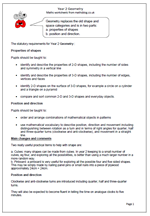Geometry replaces the old shape and space categories and is in two parts:

a. properties of shapes             b. position and direction.

The statutory requirements for Year 2 Geometry :

Properties of shapes

Pupils should be taught to:

•    identify and describe the properties of 2-D shapes, including the number of sides and symmetry in a vertical line

•    identify and describe the properties of 3-D shapes, including the number of edges, vertices and faces

•    identify 2-D shapes on the surface of 3-D shapes, for example a circle on a cylinder and a triangle on a pyramid

•    compare and sort common 2-D and 3-D shapes and everyday objects.

Position and direction

Pupils should be taught to:

•    order and arrange combinations of mathematical objects in patterns

•    use mathematical vocabulary to describe position, direction and movement including distinguishing between rotation as a turn and in terms of right angles for quarter, half and three-quarter turns (clockwise and anti-clockwise), and movement in a straight line.